# Heuristic and exact solutions to the inverse power index problem for small voting bodies

## Abstract

Power indices are mappings that quantify the influence of the members of a voting body on collective decisions a priori. Their nonlinearity and discontinuity makes it difficult to compute inverse images, i.e., to determine a voting system which induces a power distribution as close as possible to a desired one. The paper considers approximations to this inverse problem for the Penrose-Banzhaf index by hill-climbing algorithms and exact solutions which are obtained by enumeration and integer linear programming techniques. They are compared to the results of three simple solution heuristics. The heuristics perform well in absolute terms but can be improved upon very considerably in relative terms. The findings complement known asymptotic results for large voting bodies and may improve termination criteria for local search algorithms.

This is a preview of subscription content, access via your institution.

1. See Felsenthal and Machover (1998) or Laruelle and Valenciano (2008) for overviews.

2. Non-deterministic rules such as random dictatorship or also random quota rules (Dubey and Shapley 1979, Sect. 5) can easily solve the problem, but are generally not regarded as satisfactory.

3. We thank an anonymous referee for pointing out to us that Laruelle and Widgrén (1998, Sect. 6) contains the earliest published specification of an iterative algorithm for solving the inverse problem. This algorithm has recently been evaluated and improved by De Nijs and Wilmer (2012). Lucas (1992, p. 44) is one of the first to describe the basic approach to implementing a target PBI vector by a suitable voting rule; while Nurmi (1982, p. 206f) seems to be the first to state this institutional design problem and to discuss its relevance.

4. The case in which the relative weights of some voters do not vanish as n→∞—so that a few large voters or atomic players stick out in an “ocean” of infinitesimal voters (defining a so-called oceanic game)—reduces to the analysis of a modified voting body v′ for the PBI. It involves only the atomic voters and a quota q′ which is obtained from q by subtracting half of the aggregate weight of the infinitesimal voters (see Dubey and Shapley 1979, Sect. 9). If the number of atomic voters is small then the corresponding inverse problem is exactly of the type investigated here.

5. All minimal winning and hence all winning coalitions in a proper simple game have non-empty intersection. So the considered example v is not proper. We do not rule out improper games. They can play a meaningful role even in majoritarian democratic systems, e.g., if a sufficiently big parliamentary minority can install a special investigation committee or call for a referendum.

6. But each complete simple game and even each simple game can be represented as the intersection of 1≤k<∞ weighted voting games. The minimal number k of weighted voting games is called the dimension of the simple game in question (see, e.g., Taylor and Zwicker 1999; Deĭneko and Woeginger 2006).

7. Specifically, one sets $$\tilde{v}(S)=1$$ if and only if $$\sum_{U\subseteq\{ k+1,\dots ,n\}} v(T\cup U)\ge\frac{2^{n-k}}{2}$$. So the coalition T of major players—and hence all supersets S′=TU that are obtained by adding different coalitions U of “minor” players—is winning in $$\tilde{v}$$ if and only if a majority of the latter coalitions S′ are winning in v.

8. Moreover, we have $$\tilde{v}\in\mathcal{C}$$ if $$v\in\mathcal{C}$$ and $$\tilde{v}\in\mathcal{W}$$ if $$v\in\mathcal{W}$$, i.e., the construction respects completeness or weightedness of the simple game in question.

9. Such artificially constructed target vectors β n, for which Alon and Edelman’s results have bite, may not be of much practical relevance. But they indicate the problems of requiring any fixed level of accuracy in the stopping rule of a local search algorithm. Moreover, one can conceive of real-world enterprises in which the majority and minority partners indeed seek to split voting power 3:1 and want to render all n−2 other stakeholders null players.

10. A key technical requirement is that $$w_{j} \ll\sqrt{\sum w_{i}^{2}}$$ for all jN, i.e., $$w_{j}\sqrt{n}$$ is sufficiently smaller than the quadratic mean of the weights $$\sqrt{\frac{1}{n}\sum w_{i}^{2}}$$.

11. The expected value of the p-specific optimal quotas q (p) for a particular (e.g., Dirichlet) distribution of p, of course, need not coincide with the quota that is optimal when p is treated as a random variable. Stochastic optimization techniques are likely to yield a somewhat better q-heuristic than the one suggested by Słomczyński and Życzkowski (2011).

12. A minimal integer representation of a weighted voting game has the advantage that the PBI and other power indices can be computed particularly quickly.

13. Consideration of a similar variation of d broadly confirms the comparisons based on d 1, $$d_{1}'$$, and d .

14. The additional constraints (1) and (2) which ensure v’s weightedness in our ILP formulation considerably slow down the computations (because the so-called integrality gap increases). In contrast, the analogous constraints which ensure completeness impose useful structure on the problem compared to unrestrained optimization in $$\mathcal{S}$$. This explains, e.g., why an exact solution can be reported for n=10 in Table 4 in the $$\mathcal{C}$$-column but not in the $$\mathcal{S}$$ and $$\mathcal{W}$$-columns.

15. Note that the three metrics behave differently when, e.g., distance between (1,0,…,0) and $$(\frac{1}{n}, \ldots, \frac{1}{n})\in\Delta (n-1)$$ is considered for increasing n. Deviations should, therefore, be compared only within and not across tables.

16. Step size s has to be chosen with care: the number of grid points can be intractably great already for small n if s is too small. But a larger s induces a coarser grid of feasible target vectors. This becomes more and more problematic as n increases because of the corresponding natural decrease of an individual voter’s relative power (on average equal to 1/n). Choosing s=0.25, for instance, would result in the four grid points (0.5,0.25,0.25), (0.5,0.5,0), (0.75,0.25,0), and (1,0,0) for n=3. And G n would contain merely five grid points for any n≥4: (0.25,0.25,0.25,0.25,0,…,0), (0.5,0.25,0.25,0,…,0), (0.5,0.5,0,…,0), (0.75,0.25,0,…,0), and (1,0,…,0).

17. The computation times behind the unavoidable errors in Table 5 ranged from less than 1 s or 40 m for the n=5 and n=7 rows, respectively, to 14 h for the n=8 row and 31 h for n=20. Quite some time is spent on approximating the exact solution of the inverse problem. Times for the heuristic in, e.g., Table 7 were only 1 s, 15 s, 50 m, and 3 h, respectively. A sample of 10000 grid points represents a reasonable compromise between precision and computational effort. Raising the sample size to 100000 would, e.g, have produced the median, average and quantile entries (0.0041,0.0045,0.0027,0.0025,0.0020) for the d 1-metric and n=12; lowering it to 1000 would have resulted in (0.0042,0.0047,0.0028,0.0026,0.0018).

18. The construction is inspired by a sequence of weighted voting games to which the Penrose limit theorem does not apply even though every voter’s relative weight vanishes as n→∞. Namely, $$\{(\frac{1}{2};w^{n})\}_{n\in \mathbb {N}}$$ belongs to the class of problematic chains alluded to on p. 3 (cf. Lindner and Owen 2007).

19. An example for n=6 is given by the following set of minimal winning coalitions: {2,4,5,6}, {2,3,4,5}, {1,3,5,6}, {1,3,4,5}, {1,2,4,6}, and {1,2,3,5}, which attains the PBI vector $$\frac{1}{44} (8,8,8,8,8,4 )=\frac {1}{11}(2,2,2,2,2,1)$$.

20. We remark that we have imposed suitable equivalence classes of voters in the optimizations for n≥15 in order to reduce the computational burden. Voters i and j are in the same equivalence class if v(U)=v(U∪{j}∖{i}) for all coalitions U with iU and jU. This is more restrictive than requiring identical PBI values of i and j only.

21. Details on the simple but tedious computations are provided in Appendix B.

22. Interestingly, one can easily linearize the analogous inverse problem for the Shapley-Shubik power index (SSI). So even though the PBI is easier to compute than the SSI, the corresponding inverse problem is more difficult.

23. The description focuses on finding the minimal distance α . A game v Γ with B(v )=α can straightforwardly be obtained from the solution to the feasibility problem 〈β,Γ,d(⋅),α 〉.

## References

• Alon, N., & Edelman, P. H. (2010). The inverse Banzhaf problem. Social Choice and Welfare, 34(3), 371–377.

• Aziz, H., Paterson, M., & Leech, D. (2007). Efficient algorithm for designing weighted voting games. In IEEE international, multitopic conference, 2007, INMIC 2007 (pp. 1–6). Available at http://eprints.dcs.warwick.ac.uk/1547/.

• Banzhaf, J. F. (1965). Weighted voting doesn’t work: a mathematical analysis. Rutgers Law Review, 19(2), 317–343.

• Carreras, F., & Freixas, J. (1996). Complete simple games. Mathematical Social Sciences, 32(2), 139–155.

• Chang, P.-L., Chua, V. C., & Machover, M. (2006). L.S. Penrose’s limit theorem: tests by simulation. Mathematical Social Sciences, 51(1), 90–106.

• De Nijs, F., & Wilmer, D. (2012). Evaluation and improvement of Laruelle-Widgrén inverse Banzhaf approximation. Mimeo. Available at http://arxiv.org/abs/1206.1145.

• Deĭneko, V. G., & Woeginger, G. J. (2006). On the dimension of simple monotonic games. European Journal of Operational Research, 170(1), 315–318.

• Dubey, P., & Shapley, L. (1979). Mathematical properties of the Banzhaf power index. Mathematics of Operations Research, 4(2), 99–131.

• Fatima, S., Wooldridge, M., & Jennings, N. (2008). An anytime approximation method for the inverse Shapley value problem. In Proceedings of the 7th international conference on autonomous agents and multi-agent systems, Estoril, Portugal (pp. 935–942). Available at http://eprints.ecs.soton.ac.uk/15131.

• Felsenthal, D., & Machover, M. (1998). The measurement of voting power—theory and practice, problems and paradoxes. Cheltenham: Edward Elgar.

• Freixas, J., & Kurz, S. (2011). On minimal integer representations of weighted games. Mimeo. Available at http://arxiv.org/abs/1103.0868.

• Freixas, J., & Molinero, X. (2009). On the existence of a minimum integer representation for weighted voting systems. Annals of Operations Research, 166, 243–260.

• Freixas, J., & Molinero, X. (2010). Weighted games without a unique minimal representation in integers. Optimization Methods & Software, 25(2), 203–215.

• Grilli di Cortona, P., Manzi, C., Pennisi, A., Ricca, F., & Simeone, B. (1999). SIAM monographs on discrete mathematics and applications. Evaluation and optimization of electoral systems. Philadelphia: SIAM.

• Isbell, J. R. (1956). A class of majority games. Quarterly Journal of Mathematics, 7(1), 183–187.

• Kaniovski, S. (2008). The exact bias of the Banzhaf measure of power when votes are neither equiprobable nor independent. Social Choice and Welfare, 31(2), 281–300.

• Keijzer, B. d. (2009). On the design and synthesis of voting games. Master’s thesis, Delft University of Technology.

• Keijzer, B. d., Klos, T., & Zhang, Y. (2010). Enumeration and exact design of weighted voting games. In Proceedings of the 9th international conference on autonomous agents and multiagent systems (Vol. 1, pp. 391–398).

• Kirsch, W., Słomczyński, W., & Życzkowski, K. (2007). Getting the votes right. European Voice, (3–9 May), 12.

• Kurth, M. (2008). Square root voting in the Council of the European Union: rounding effects and the Jagiellonian compromise. Available at http://arxiv.org/abs/0712.2699.

• Kurz, S. (2012a). On minimum sum representations for weighted voting games. Annals of Operations Research, 196(1), 361–369.

• Kurz, S. (2012b). On the inverse power index problem. Optimization, 61(8), 989–1011.

• Kurz, S., Maaser, N., & Napel, S. (2012). On the egalitarian weights of nations. Mimeo, University of Bayreuth.

• Laruelle, A., & Valenciano, F. (2008). Voting and collective decision-making. Cambridge: Cambridge University Press.

• Laruelle, A., & Widgrén, M. (1998). Is the allocation of power among EU states fair? Public Choice, 94(3–4), 317–340.

• Leech, D. (2002a). Designing the voting system for the EU Council of Ministers. Public Choice, 113(3–4), 437–464.

• Leech, D. (2002b). Voting power in the governance of the international monetary fund. Annals of Operations Research, 109(1), 375–397.

• Leech, D. (2003). Power indices as an aid to institutional design: the generalised apportionment problem. In M. J. Holler, H. Kliemt, D. Schmidtchen, & M. E. Streit (Eds.), Jahrbuch für Neue Politische Ökonomie (Vol. 22). Tübingen: Mohr Siebeck.

• Lindner, I., & Machover, M. (2004). L.S. Penrose’s limit theorem: proof of some special cases. Mathematical Social Sciences, 47(1), 37–49.

• Lindner, I., & Owen, G. (2007). Cases where the Penrose limit theorem does not hold. Mathematical Social Sciences, 53(3), 232–238.

• Lucas, W. F. (1992). HistoMAP module: Vol. 19. Fair voting: weighted votes for unequal constituencies. Lexington: COMAP.

• Neyman, A. (1982). Renewal theory without replacements. Annals of Probability, 10(2), 464–481.

• Nurmi, H. (1982). The problem of the right distribution of voting power. In M. J. Holler (Ed.), Power, voting, and voting power. Würzburg: Physica-Verlag.

• Pennisi, A., Ricca, F., Serafini, P., & Simeone, B. (2007). Amending and enhancing electoral laws through mixed integer programming in the case of Italy. In E. Yashin (Ed.), Proceedings of the 8th international conference on economic modernization and social development. Moscow: HSE.

• Penrose, L. S. (1946). The elementary statistics of majority voting. Journal of the Royal Statistical Society, 109(1), 53–57.

• Penrose, L. S. (1952). On the objective study of crowd behaviour. London: Lewis

• Ricca, F., Scozzari, A., Serafini, P., & Simeone, B. (2012). Error minimization methods in biproportional apportionment. Top, 20(3), 547–577.

• Shapley, L. S., & Shubik, M. (1954). A method for evaluating the distribution of power in a committee system. The American Political Science Review, 48(3), 787–792.

• Słomczyński, W., & Życzkowski, K. (2006). Penrose voting system and optimal quota. Acta Physica Polonica B, 37, 3133–3143.

• Słomczyński, W., & Życzkowski, K. (2007). From a toy model to the double square root system. Homo Oeconomicus, 24(3/4), 381–399.

• Słomczyński, W., & Życzkowski, K. (2011). Square root voting system, optimal threshold and π. Available at http://arxiv.org/abs/1104.5213.

• Taylor, A. D., & Zwicker, W. S. (1999). Simple games. Princeton: Princeton University Press.

## Author information

Authors

### Corresponding author

Correspondence to Sascha Kurz.

We thank Nicola Maaser and Friedrich Pukelsheim for constructive comments on an earlier version. A dispute with one of the referees—about the so-called “Penrose limit theorem” and whether Lionel S. Penrose can be said to have suggested asymptotic proportionality of voting weight and power for n→∞—could not be resolved with mutual agreement. We are still grateful to both anonymous referees for their helpful suggestions. Napel acknowledges the generous hospitality of the Max Planck Institute of Economics, Jena, where parts of this paper were written.

## Appendices

### Appendix A: ILP formulation for the inverse Penrose-Banzhaf index problem

Even though stating the inverse power index problem as an optimization problem is trivial (see (3)), coming up with an implementable formulation for finding an exact solution is not. Voting systems are discrete objects, and so some kind of discrete optimization is needed. Exhaustive enumeration (see Keijzer et al. 2010) is limited at best to n≤9 (see Table 1). A much more tractable alternative is to describe the set of feasible binary voting systems by integer variables and to use some of the available optimization software packages. These allow significantly larger numbers of variables when dealing with linear rather than non-linear (mixed) integer optimization problems. So it is unfortunate that problem (3) cannot directly be translated into a linear problem for the Penrose-Banzhaf index.Footnote 22 The “work-around”, which has first been suggested by Kurz (2012b) and is adopted here, is to use ILP techniques in order to merely find out whether some binary voting system v exists whose PBI vector B(v) is at most a specified distance α apart from the target β. This feasibility problem can be solved much more easily than the underlying minimization problem. Still, one can iteratively determine the exact solution of (3) by varying α.

We will mostly confine our description to the case of measuring distance by the d 1-metric. Adaptations to the $$d_{1}'$$ or d -metric are straightforward. They involve heterogeneous coefficients in inequality (19) below for $$d_{1}'$$, and neither i-subscripts in (16)–(18) nor a summation in (19) for d .

The PBI vector (1,0,…,0) of a dictator has at most a d 1-distance of 2 from any normalized power distribution (summing to 1). This is in fact the worst case, and the minimal achievable deviation α must lie inside the interval [l 1,u 1], where l 1=0 and u 1=2. In each iteration t=1,…,T of the algorithm we will check whether α=(u t l t )/2 is a feasible distance between target β and the PBI values generated by the considered class of voting systems. If so, we set u t+1=α and leave l t+1=l t unchanged; otherwise we update l t+1=α and leave u t+1=u t unchanged. In each iteration the length of the interval [l t ,u t ] shrinks by a factor of 2. Since the total number of swings in an n-player voting game lies between n and $$m\binom{n}{ m}<n2^{n}$$ where $$m= \lfloor\frac {n}{2} \rfloor+1$$ (see, e.g., Felsenthal and Machover 1998, Sect. 3.3), two distinct PBI vectors differ, both in the d 1 and the d -metric, by at least $$(\frac{1}{n2^{n}} )^{2}$$. A finite number T of iterations are, therefore, sufficient for obtaining a solution. More specifically, O(n) bisections on α are needed before $$u_{t}-l_{t}\le (\frac {1}{n2^{n}} )^{2}$$ and further improvements become theoretically impossible.

A pseudo-code description of this bisection approach reads as follows:Footnote 23

• Input: desired power index vector β, class of binary voting systems Γ, metric d(⋅)

• Output: minimum d-distance α between β and PBI vectors induced by Γ

• l 1=0

• u 1=2

• α =2

• $$\varepsilon= (\frac{1}{n2^{n}} )^{2}$$

• t=1

• while u t l t >ε

• $$\alpha=\frac{u_{t}-l_{t}}{2}$$

• t=t+1

• solve feasibility problem 〈β,Γ,d(⋅),α

• if vΓ such that d(B(v)−β)≤α exists

• then

• u t =d(B(v)−β), α =u t

else

• l t =α

end if

• end while

• return α

The feasibility problemβ,Γ,d(⋅),α〉 consists of verifying whether there exists a voting system vΓ such that d(B(v),β)≤α. The following ILP formulation describes it for $$\varGamma=\mathcal{S}$$ and the d 1-metric. Adaptations to $$\mathcal{C}$$ or $$\mathcal{W}$$ and $$d_{1}'(\cdot)$$ or d (⋅) involve further variables and (modified) constraints, but are otherwise very similar:(7)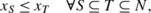(8)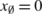(9)(10)(11)(12)(13)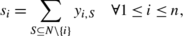(14)(15)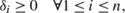(16)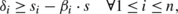(17)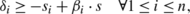(18)(19)

The binary variables x S define a Boolean function v via v(S)=x S ; inequalities (7)–(10) ensure that they represent a simple game. The binary auxiliary variables y i,S =x S∪{i}x S which are introduced in (11)–(12) for all iN and ∅⊆SN∖{i} satisfy y i,S =1 if and only if coalition S is a swing for voter i, i.e., contributes 1/2n−1 to $$B_{i}'(v)$$. They are used in order to determine the number of swings $$s_{i}=2^{n-1}\cdot B_{i}'(v)$$ for each player i in Eq. (14). The total number of swings $$s=\sum_{i=1}^{n}s_{i}$$ is defined in Eq. (15). Based on this total number, the individual deviation δ i =|s i β i s| from the target number of swings is captured by inequalities (17) and (18). The feasibility of a d 1-distance α is then finally checked by introducing constraint (19). Namely, a simple game $$v\in\mathcal{S}$$ whose PBI has d 1-distance of α or less exists if and only if the feasible set defined by (7)–(19) is non-empty.

The answer to whether this is the case—and, as a by-product, some $$v\in\mathcal{S}$$ with distance at most α—can be obtained by feeding (7)–(19) into a standard ILP software package in the required format. We have used IBM ILOG CPLEX 12.4 and the hardware described in Sect. 5.

### Appendix B: Analytical PBI calculations

This appendix presents some technical details on the PBI computations for the sequence of weighted voting games {v n} n∈ℕ with

$$v^n=\bigl(q^n;w^n\bigr)=(2n-a-4; \underbrace{3,\ldots, 3}_{a \,\mathrm{threes}}, \underbrace{2,\ldots, 2}_{n-a-1 \,\mathrm{twos}},1),$$

which is considered in the final paragraphs of Sect. 5.3. Our first lemma determines the number of swings in v n for each voter i=1,…,n, that is, the cardinality of set {SN∖{i}:v n(S∪{i})−v n(S)=1}, as a function of a and n.

### Lemma 1

The numbers of swings in v n are 2na−2, 2na−4, and a for all voters with weight 3, 2, and 1, respectively.

### Proof

It is convenient to exploit the fact that for any $$v\in \mathcal{S}$$ the number of voter i’s swings in v and in the dual game $$v'\in\mathcal{S}$$ which is obtained by setting v′(S)=1−v(S) for all SN must coincide. So instead of v n consider the game v n′ which involves identical weights but quota q′=4 instead of 2na−4. Referring to winning and losing coalitions in v n′ we have:

1. (i)

A voter i with w i =3 renders a losing coalition SN∖{i} winning by joining if either |S|=1 or S={j,k} with w j =2 and w k =1. There are n−1 coalitions of the former and na−1 coalitions of the latter type, amounting to 2na−2 swings altogether.

2. (ii)

A voter i with w i =2⊆N∖{i} renders a losing coalition S winning by joining if either S={w j } with w j =3 or 2, or S={j,k} with w j =2 and w k =1. There are a+(na−2) coalitions of the former and na−2 coalitions of the latter type, amounting to 2na−4 swings altogether.

3. (iii)

Voter n with w n =1 renders a losing coalition SN∖{i} winning by joining if S={j} with w j =3. There are a such coalitions.

□

Writing ⌊x⌋ to denote the largest integer not greater than x, and x mod y to denote the integer remainder when x is divided by y, we have the following finding for distances in the d 1-metric:

### Lemma 2

Choose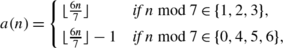and consider

$${v^n} = (2n-a-4;\underbrace{3,\ldots, 3}_{a(n) \,\mathrm{threes}}, \underbrace{2,\ldots, 2}_{n-a(n)-1 \,\mathrm{twos}},1).$$

Then

$$\lim_{n\to\infty} n\cdot \bigl(d_1\bigl(B\bigl({v^n} \bigr),\beta^n\bigr) \bigr)=\frac{1}{2}.$$

### Proof

Suppose that n mod 7=0, i.e., n=7k+0 for some k∈ℕ. Then a=6k−1 and Lemma 1 yields swing numbers of 2⋅7k−(6k−1)−2, 2⋅7k−(6k−1)−4, and 6k−1 for the three voter types, respectively. This implies a total number of

$$(6k-1) (8k-1)+(n-6k) (8k-3)+(6k-1)=56k^2-11k$$

swings, and hence a PBI vector of

$$B\bigl(v^{7k}\bigr)=\frac{1}{56k^2-11k} (\underbrace{8k-1, \ldots, 8k-1}_{6k-1 \,\mathrm{times}}, \underbrace{8k-3, \ldots, 8k-3}_{k \, \mathrm{times}}, 6k-1 ).$$

This yields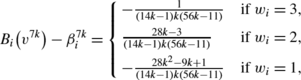and summing the absolute values of these figures up for the 6k−1 voters with weight 3, the k voters with weight 2, and the final voter n one obtains

$$\bigl\Vert B_i\bigl(v^n\bigr)-\beta^n \bigr\Vert_1= \frac{2(28k-3)}{(14k-1)k(56k-11)}$$

in case of n=7k. This number and results of the similarly tedious computations when n mod 7=1,…,6 are summarized in Table 11. For each of the seven cases one easily sees that the deviations tend to $$\frac{1}{14k}$$, which is equivalent to $$\frac{1}{2n}$$.

□

In case of the d -metric, choose

$$a(n)= \bigl\lfloor(n+1)/3 \bigr\rfloor+ \lfloor n/3 \rfloor-1.$$

In each corresponding game v n (see Lemma 2) roughly two thirds of the players have weight 3 each, roughly one third have weight 2, and a single player has weight 1. The games v n result in very good solutions of the inverse problem for n<8 and the best ones we could find for n≥8. Using this in order to obtain an upper bound one can verify the following result in perfect analogy to Lemma 2:

### Lemma 3

The weighted voting game $$v^{***}\in\mathcal{W}$$ whose PBI minimizes d -distance to β n satisfies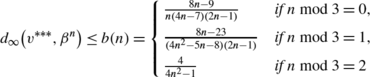for n≥8.

Note that the indicated bound tends to $$\frac{1}{n^{2}}$$, i.e., $$\lim_{n\to\infty} {b(n)} /{ \frac{1}{n^{2}}}=1$$.

## Rights and permissions

Reprints and Permissions

Kurz, S., Napel, S. Heuristic and exact solutions to the inverse power index problem for small voting bodies. Ann Oper Res 215, 137–163 (2014). https://doi.org/10.1007/s10479-012-1293-0

• Published:

• Issue Date:

• DOI: https://doi.org/10.1007/s10479-012-1293-0

### Keywords

• Electoral systems
• Simple games
• Weighted voting games
• Square root rule
• Penrose limit theorem
• Penrose-Banzhaf index
• Institutional design